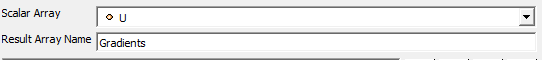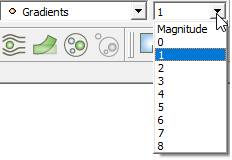# calculating the vicious part of drag

I have an OpenFOAM simulation wich gives me the p, Ux, Uy, Uz and T coutures. I want to calculate the total drag including the hydrostatic and viscous elements, on some surfaces. I have followed this tutorial and I’m able to calculate the hydrostatic part. however all of the examples I see on the internet ignore the vicious part. Although in the end it can be shown that it is negligible but I have to show it in my study.
There is a filter called Gradient Of Unstructured DataSet and if I apply it on U:It generates 9 elements as expected:The first issue is that I don’t know which element refers to ∂Uz/ ∂x which in my case should be the vicious stress. It must be 2 or 6 but not sure.
the second issue is when I want to apply the calculator filter to calculate 𝜂∂Uz/ ∂x in Attribute section>cell data there are not gradients, but they are in the point Data:

I would appreciate if you could help me solve this issue. maybe if there are any examples for calculating viscous stresses on an object that could give me some clue. Thank sin advance.

From the filter “The ordering for the output gradient tuple will be {du/dx, du/dy, du/dz, dv/dx, dv/dy, dv/dz, dw/dx, dw/dy, dw/dz} for an input array {u, v, w}”.

The filter computes cell gradients or point gradients base on whether the array chosen for gradient computation was cell data or point data. Since your U is a point data array, the gradients are computed per point. Hence the Calculator filter can only use gradients in the expression when computing a new point field.

1 Like

To address the second issue, you might try using the Compute Derivatives filter instead of the Gradient Of Unstructured DataSet filter. It performs similar operations, but it places the gradient/derivative as cell data instead of point data.

1 Like

Thanks a lot. I did not know about the Compute Derivatives filter. comparing the results with Gradient, they seem identical.